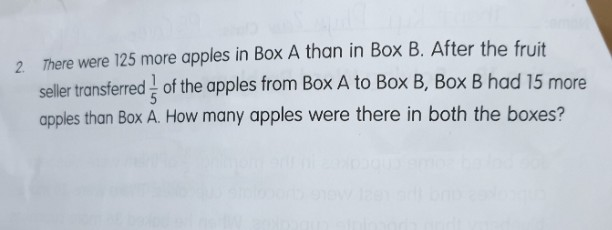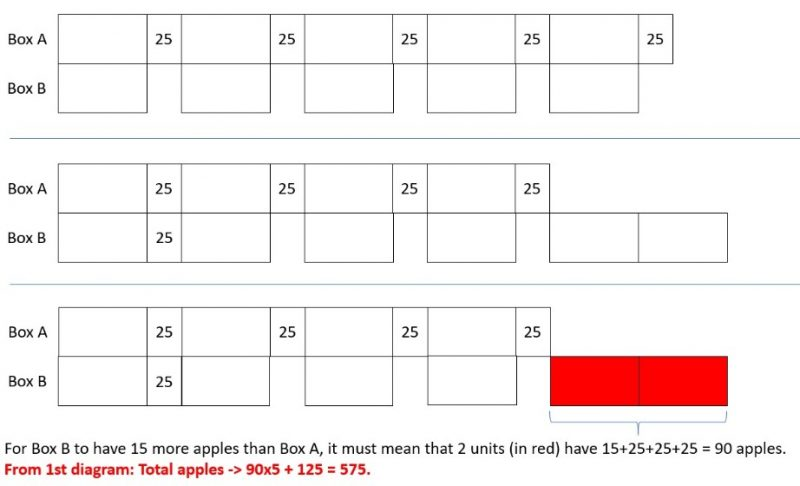# QuestionHi,

Can anyone help us with this?

Would be awesome if you could kindly show me how to do it step by step.

Thanks for your time.

Cheers

assume box A has 5 unit,  at beginning, A = 5 unit, B = 5 unit -125,

after give 1/5, which is one unit,  A = 4 unit, B = 6 unit -125, and now B has 15 more apples, so 2 unit = 125+15, one unit is 70, box A has 350 apples

1 Reply 1 Like

Hi,

Thanks for the reply.

I am confused at  2 unit = 125+15.

Can you explain why? Thanks a lot.

2 Replies 0 Likes

That’s because after the transfer, A has 4 units, B has 6 unit -125. Since B has 15 more,
6 unit – 125 = 4 unit + 15
2 unit = 125 + 15

A = 4u  b = 6u -125, u got this part right?

and B has 15 more, that mean A + 15 = B

so  4u + 15 = 6u -125, we can write as 4u + 15 = 4u + 2u -125

so 15 = 2u -125,  although this is somehow like an equation, you can draw the model to get it, I cannot draw it here.

0 Replies 1 Like

Box A : (5 units + 125) – (1/5) x (5 units + 125) = 4 units + 100
Box B : 5 units + (1/5) x (5 units + 25) = 6 units + 25

6 units + 25 = 4 units + 100 + 15
1 unit = 90/2 = 45
5 units + 125 + 5 units or 4 units + 100 + 6 units + 25
= 10 units + 125
= 10 x 45 + 125 = 575

Ans : 575 apples.

0 Replies 0 Likes0 Replies 0 Likes